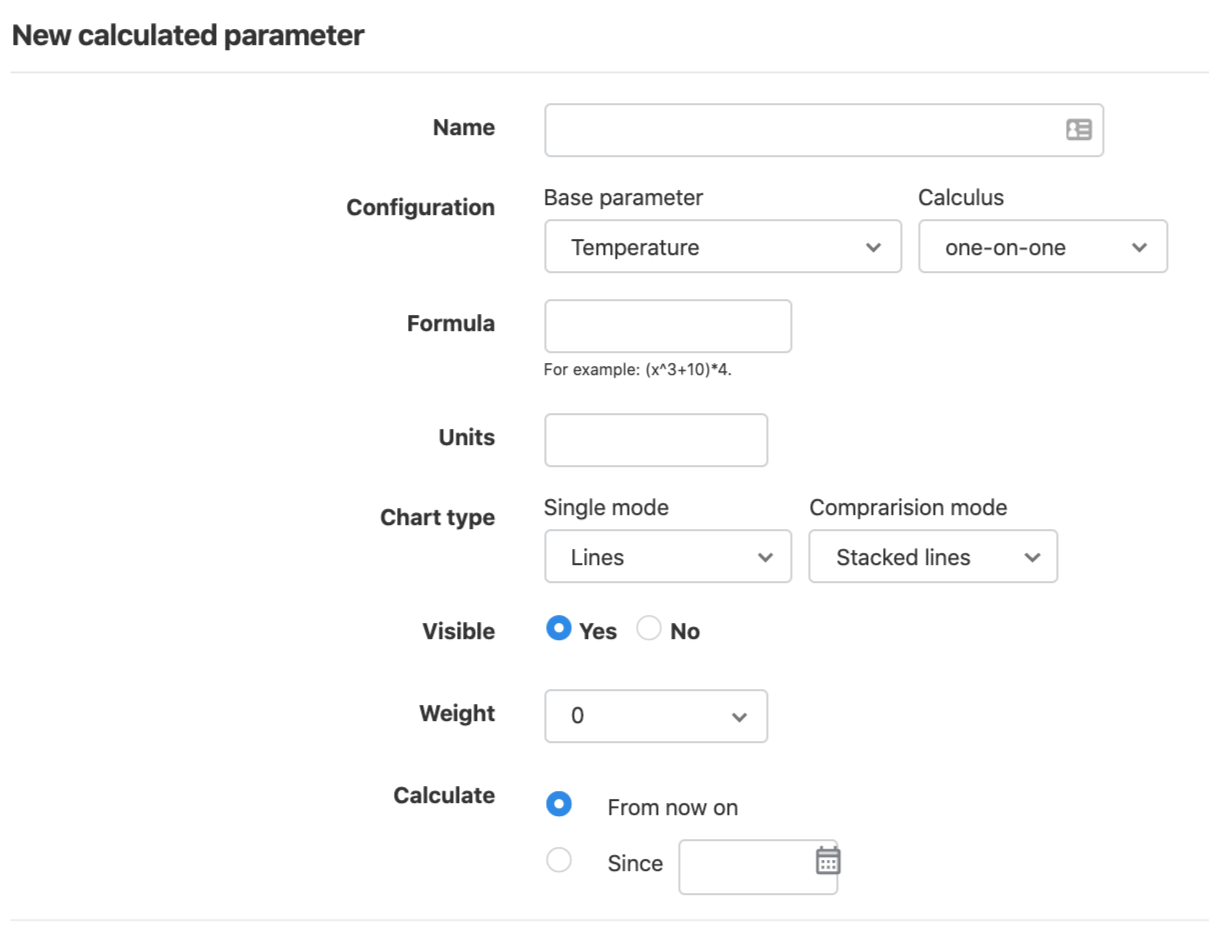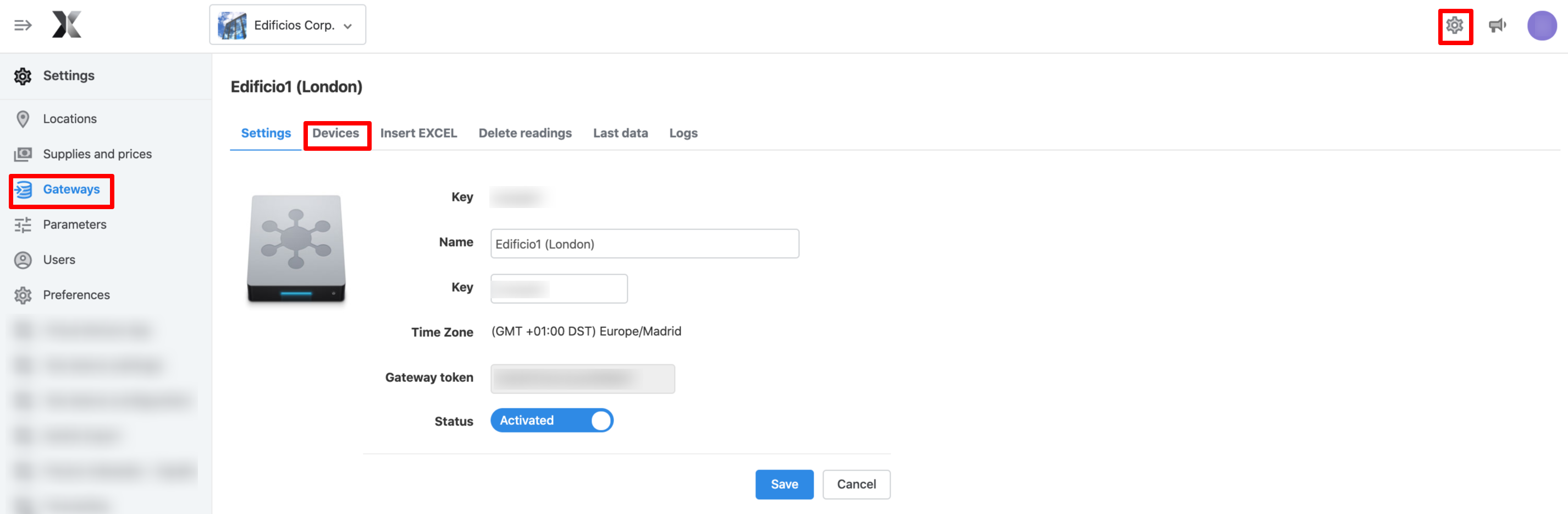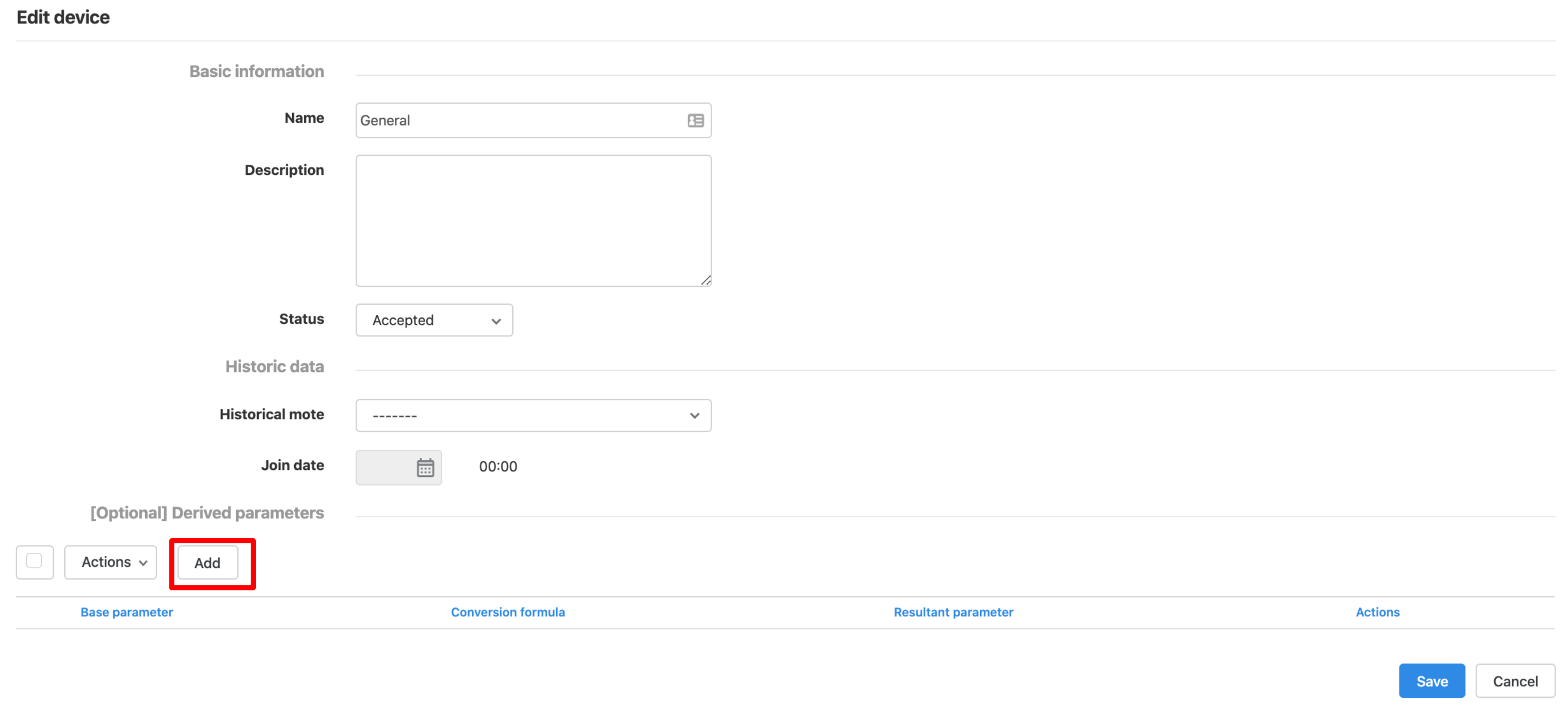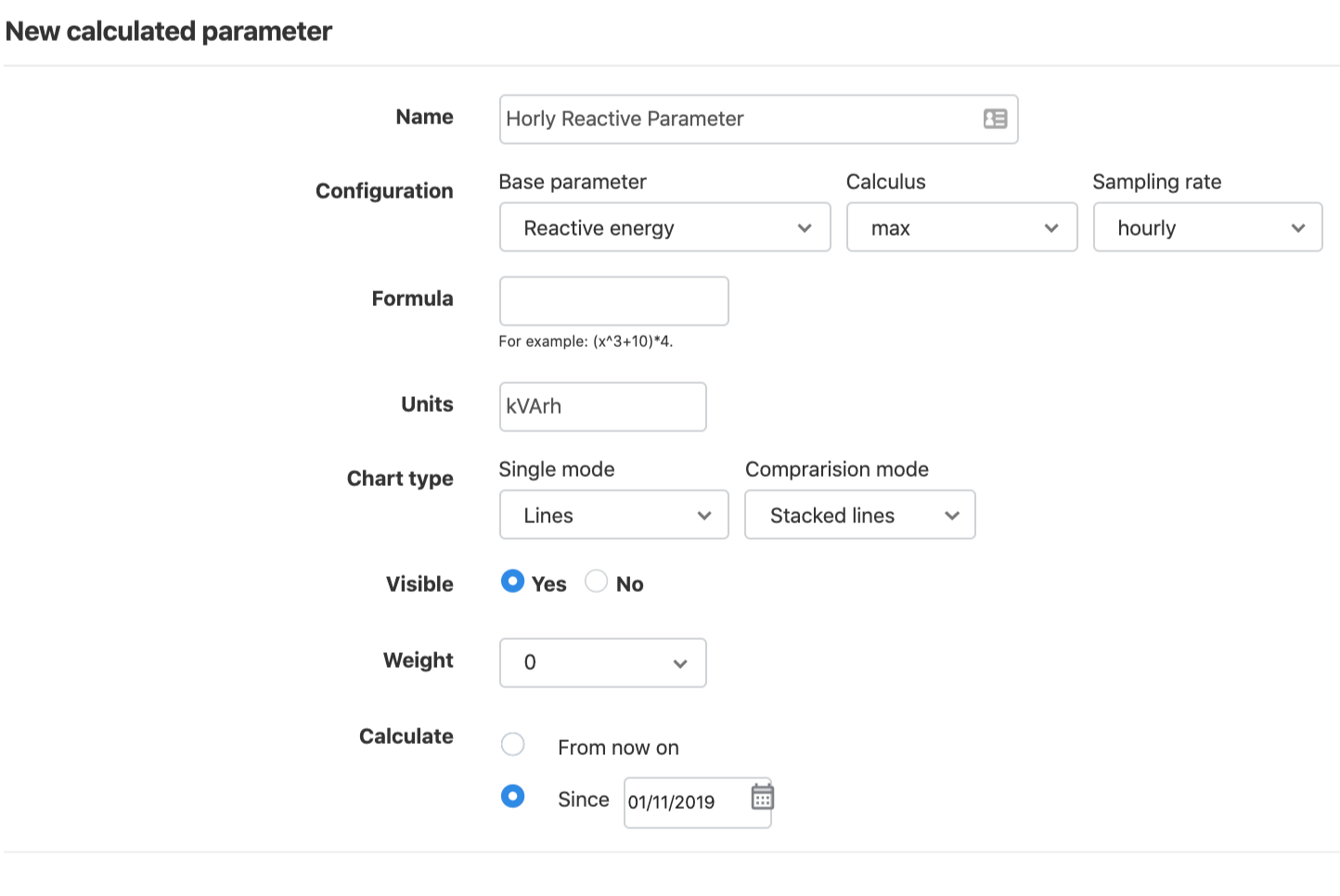# Derived and Calculated Parameters

In this article, it is explained how to create a calculated and a derived parameter, as well as how to create the hourly reactive energy parameter:

### Create a calculated parameter

1. To create a new calculated parameter, go to "Parameters" screen on "Settings" menu.2. If you want to create a new calculated parameter, go to the "Calculated Parameters" and click on "New calculated parameter". The options are identical to those of the basic parameters except set point. You should:
• Introduce a Name for the new parameter.
• Select a Base parameter. The calculated parameter will be created from a base parameter.
• If you don't have any data in the base parameter, the calculated parameter will not have any data either.
• Then, select one of the supported calculus [one-by-one, cumulative, average, max, min] and sampling frequency [ hourly, daily, weekly, monthly]
• One-by-one: Formula calculation will be applied.
• Cumulative: A basic parameter integral will be done.
• Average: Geometric average calculation will be performed, based on the period selected in the sampling rate field.
• max / min: Maximum or minimum points for the selected period based on the sampling rate will be done.
• Formula: This field allows you to add an additional formula to be performed on the calculated parameter.
• Units: Units from the formula.
• Weight: Indicates the level of importance of the parameter, in order to list the parameters by the level of priority.
• Chart type: Which type of chart you want to generate (columns or rows). Also, you have to indicate if you make it visible or not.
• Calculate from: Select the date from which the parameter has to be calculated. The option "Since" implies that the software will go back in time and calculate the new variable from that moment onwards.
• Calculated parameters can be Edited, Deleted or Recalculated.You can view and analyse your new parameters in the Analysis screen, in the "By Device" or Advanced Analytics menus or in "By parameter" Widgets.

Calculated parameters are free!

### Create Derived parameters

Following this manual, you will be able to convert a parameter into another one, simply doing a bypass or converting it with different correction factors.

This is useful when the gateway does not send data into the software with the correct parameter identifiers or when you are doing Gas Metering. These are the steps you should follow to create a derived parameter:

2. Go to "Settings" and click on "Gateways" menu.
3. Select a gateway and click on the "devices" tab.4. Edit the device in which you would like to create a derived parameter.5. From any device, you will be able to derive as many different parameters as you want. For example, from a Gas Massflow (m3), you can get Gas normalised massflow (Nm3) and Gas Energy (kWh).
6. On the form, focus on [Optional] Derived parameters.7.   Select a "Base Parameter", a conversion formula if needed and the "Resultant parameter".
• Example: Derived parameter - If you need to do some operations during the bypass, use the following configuration (with your own correct factors):8. Then save and you will have created the derived parameter.

9. When new data arrives for the base parameter, you will find that the derived parameter is listed and ready to be accepted in the Gateway's Devices tab. Click in Parameters on the list of devices and the list of parameters available for that device will pop up.

*NOTE: derived parameters cannot be calculated backwards, i.e., once a derived parameter is created, when the next value of the basic parameter is inserted, then the first value for the derived parameter will appear. If you would like to have the values before the date of the derived parameter configuration, you should re-insert the data for the base parameter by manually uploading the data through an Excel file.

Another option would be to create the derived parameters using the Virtual Devices App. This App would allow you to calculate backwards the derived parameter. However, please note that the parameters created through this App are billable.

**NOTE: when the derived parameter is created, in the device it will appear a message saying that there is a DP pending to be accepted. You will have to accept the DP to see the derived parameter's values.

### Creating the Hourly Reactive Energy Parameter

1. Go to the "Parameters" screen in the "Settings" menu.2. Go to the "Calculated Parameters" tab, and click on "New calculated parameter" button.
3. Write the name on "Name" field: Hourly Reactive Energy.
4. From the base parameter, select Reactive energy.
5. On calculus, select "Cumulative".
6. On Sampling rate, select "Hourly".
7. Formula field can rest blank.
8. In units, write your according unit. In this case, energy comes in kWh, so reactive energy is kVArh.
9. Chart type, visible and weight fields, can be configured as shown in the image below.
10. In the field calculate from, select the starting date where you would like to start having the parameter available.